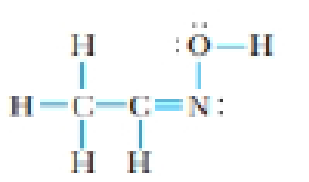Chapter 9, Problem 37GQ

Chapter
Section
Textbook Problem

The organic compound below is a member of a class known as oximes.(a) What are the hybridizations of the two C atoms and of the N atom?(b) What is the approximate C—N—O angle?

(a)

Interpretation Introduction

Interpretation:

The hybridizations of the two carbon atoms and the nitrogen atom should be identified.

Concept Introduction:

Hybridization:

• It is the mixing of valence atomic orbitals to get equivalent hybridized orbitals that having similar characteristics and energy.
• Geometry of a molecule can be predicted by knowing its hybridization.
• sp3  hybrid orbitals is produced by hybridization of single s-orbital and three p-orbital.
• sp2  hybrid orbitals is produced by hybridization between one s-orbital and two p-orbitals.
• sp  hybrid orbitals is produced by hybridization of single s-orbital and single p-orbital.

Geometry of different types of molecule with respect to the hybridizations are mentioned are mentioned below,

TypeofmoleculeHybridaizationAtomicorbitalsusedforhybridaizationGeometryAX2sp1s+1pLinearAX3,AX2Bsp21s+2pTrigonalplanarAX4,AX3B,AX2B2sp31s+3pTetrahedralAX5,AX4B,AX3B2,AX2B3sp3d1s+3p+1dTrigonalbipyramidalAX6,AX5B,AX4B2sp3d21s+3p+2dOctahedralACentralatomXAtomsbondedtoABNonbondingelectronpairsonA

Geometry of different type of molecules with respect to the number of electron pairs are mentioned below,

TypeofMoleculeNo.ofatomsbondedtocentralatomsNo.oflonepairsoncentralatomArrangementofelectronpairsMolecularGeometryAB220LinearLinearAB330TrigonalplanarTrigonalplanarAB440TetrahedralTetrahedralAB550TrigonalbipyramidalTrigonalbipyramidalAB660OctahedralOctahedral

Bond angle: The bond angle is the angle formed between three atoms at least two bonds.

Explanation

The given organic compound is,

There are four electron pairs around carbon atom. Four electron pairs will orient in a tetrahedral manner. Generally tetrahedral geometry is a result of sp3 hybridization. Therefore,

The carbon atom is sp3 hybridized.

Three electron pairs are present around the second carbon atom. An atom surrounded by three electron pairs constitutes a trigonal planar geometry. Generally trigonal planar geometry arises due to sp2 hybridization

(b)

Interpretation Introduction

Interpretation:

The approximate bond angle between CNO in the given molecule should be determined.

Concept Introduction:

VSEPR Theory:

As the name itself indicates that the basis for this theory is the electron pair that is bonded electron present in either single or double bonds or lone pair electrons, present in the valence shell tends to repel each other which then tend to be in position in order to minimize the repulsions.

Bond angle: The bond angle is the angle formed between three atoms at least two bonds.

Still sussing out bartleby?

Check out a sample textbook solution.

See a sample solution

The Solution to Your Study Problems

Bartleby provides explanations to thousands of textbook problems written by our experts, many with advanced degrees!

Get Started